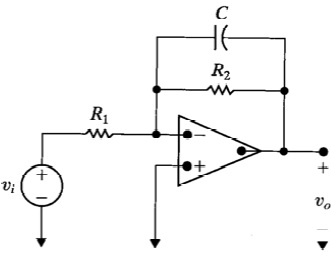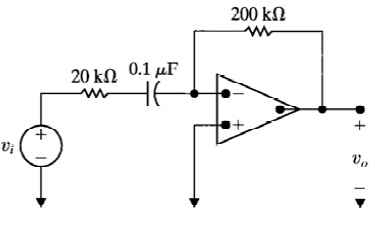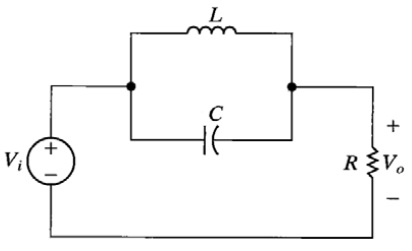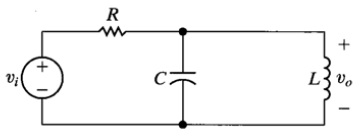+61-413 786 465

info@mywordsolution.com

## Engineering

 Civil Engineering Chemical Engineering Electrical & Electronics Mechanical Engineering Computer Engineering Engineering Mathematics MATLAB Other Engineering Digital Electronics Biochemical & Biotechnology

In submitting the problem set, provide a title page with the required information. This is followed by numbered pages showing the results for the four problems. For each of the four problems, two figures will be present. The first figure is the developed MATLAB script file (be sure to use all black text and a fixed width font like Courier) while the second figure shows the graphical results. For each figure, provide a numbered descriptive figure caption while for the m file provide appropriate comments; in the very least, the first line of  code must contain a comment line with your name, course number and semester/year. For a low pass or high pass filter, mark the critical frequency on the amplitude/magnitude plot while for a band pass or band reject filter mark the resonant (center) frequency on the amplitude/magnitude plot. (For a low/high pass filter, the critical frequency has a gain that is 3dB less than the maximum gain. For a band pass/reject filter, the resonant/center frequency occurs at the maximum or minimum gain respectively.) If necessary, move the resulting box marker so it covers the least amount of important information on the graph. ]

Note that by default, the horizontal axis for the bode command in MATLAB generates a horizontal axis in rad/sec (ω). one must have a horizontal axis in Hz (f). As such, for each filter, have the command

set(cstprefs.tbxprefs,'FrequencyUnits','Hz') just before the bode command in the m file.

problem) The transfer function for the frequency selective circuit in Figure 1 can be shown to be

Vo(s)/Vi(s) = [(-R2/R1)/ sR2C+1]

where R1=1.5kΩ, R2= 1.5kΩ and C= 0.2µF. Use MATLAB to obtain the amplitude and phase frequency response using the tf and bode commands. Show the m file used and the resulting plot. The plot must have the title “Bode plot for problem 1 by Jane Doe”. Here, Jane Doe is replaced by your name. For the Bode plot figure caption, indicate the filter type (low pass, high pass, band pass or band reject) and critical frequency (if low pass or high pass) or resonant (center) frequency (if band pass or band reject) in Hz.problem) The transfer function for the frequency selective circuit in Figure  can be shown to be

Vo(s)/Vi(s) = [-sR2C/ sR1C+1]

where R1= 20kΩ, R2= 200kΩ and C= 0.1µF. Use MATLAB to obtain the amplitude and phase frequency response using the tf and bode commands. Show the m file used and the resulting plot. The plot must have the title “Bode plot for problem 2 by Jane Doe”. Here, Jane Doe is replaced by your name. For the Bode plot figure caption, indicate the filter type (low pass, high pass, band pass or band reject) and critical frequency (if low pass or high pass) or resonant (center) frequency (if band pass or band reject) in Hz. That is, configure MATLAB so that the horizontal axis is Hz and not the default rad/s (the frequency range will be determined automatically by MATLAB).problem) The transfer function for the frequency selective circuit in Figure which is shown below can be represented as:

Vo(s)/Vi(s) = [s2RLC+R/ s2RLC+sL+R]

shown to be where R=2.2kΩ, L= 560µH and c= 5nF. Use MATLAB to obtain the amplitude and phase frequency response using the tf and bode commands. Show the m file used and the resulting plot. The plot must have the title “Bode plot for problem 3 by Jane Doe”. Here, Jane Doe is replaced by your name. For the Bode plot figure caption, indicate the filter type (low pass, high pass, band pass or band reject) and critical frequency (if low pass or high pass) or resonant (center) frequency (if band pass or band reject) in Hz. That is, configure MATLAB so that the horizontal axis is Hz and not the default rad/s (the frequency range will be determined automatically by MATLAB).problem) The transfer function for the frequency selective circuit in Figure which is shown below can be represented as:

Vo(s)/Vi(s) = [sL/ s2RLC+sL+R]

Where R=2.2kΩ, L= 560µH and c= 5nF. Use MATLAB to obtain the amplitude and phase frequency response using the tf and bode commands. Show the m file used and the resulting plot. The plot must have the title “Bode plot for problem 4 by Jane Doe”. Here, Jane Doe is replaced by your name. For the Bode plot figure caption, indicate the filter type (low pass, high pass, band pass or band reject) and critical frequency (if low pass or high pass) or resonant (center) frequency (if band pass or band reject) in Hz. That is, configure MATLAB so that the horizontal axis is Hz and not the default rad/s (the frequency range will be determined automatically by MATLAB)Electrical & Electronics, Engineering

• Category:- Electrical & Electronics
• Reference No.:- M9316

Have any Question?

## Related Questions in Electrical & Electronics

### A four-pole star-connected squirrel-cage induction motor

A four-pole, star-connected, squirrel-cage induction motor operates from a variable voltage 50 Hz three-phase supply. The following results were obtained as the supply voltage was gradually reduced with the motor running ...

Research report 1. Read 3 to 4 journal articles about digital control or industrial control, eg. one particular application, implementation aspect such as selection of sampling time, hardware etc. No text book example is ...

### Problem 1 a two-phase servomotor has rated voltage applied

Problem 1: A two-phase servomotor has rated voltage applied to its excitation winding. The torque speed characteristic of the motor with Vc = 220 V, 60 Hz applied to its control phase winding is shown in Fig.1. The momen ...

### Discuss the role of load factor on the cost of electrical

Discuss the role of load factor on the cost of electrical energy.

### Question -i a star-connected three-phase synchronous

Question - (i) A star-connected, three-phase synchronous induction motor takes a current of 10 amps from a 415 volt supply at unity power factor when supplying a steady load. If the synchronous reactance is 5 ohms/phase ...

### 1 goalin this project you will solve a non-trivial design

1 Goal In this project you will solve a non-trivial design problem explicitly using the divide-and-conquer (D&C) approach. The main reason for using the D&C approach is the ease of the design process and the streamlined ...

### Nanotechnology engineering - resonance circuits questions

Nanotechnology Engineering - Resonance Circuits Questions - Q1) A series RLC network has R = 2KΩ, L = 40mH and C = 1μF. Calculate the impedance at resonance and at one-fourth, one-half, twice, and four times the resonant ...

### Questions -problem 1 - a series rlc network has r 2 komega

Questions - Problem 1 - A series RLC network has R = 2 kΩ, L = 40 mH and C = 1μF. Calculate the impedance at resonance and at one-fourth, one-half, twice, and four times the resonant frequency. Problem 2 - Design a serie ...

### Questions -problem 1 - determine the laplace transform ofa

Questions - Problem 1 - Determine the Laplace transform of: (a) cos(ωt + θ) (b) sin(ωt + θ) Problem 2 - Obtain the Laplace transform of each of the following functions: (a) e -2t cos(3t)u(t) (b) e -2t sin(4t)u(t) (c) e - ...

### 1 a name the three major groups of contamination and

1. (a) Name the three major groups of contamination and briefly describe their physical characteristics. (b) Where do the above contamination types come from? Give one example of each. 2. Name two processes metrics which ...

• 13,132 Experts

## Looking for Assignment Help?

Start excelling in your Courses, Get help with Assignment

Write us your full requirement for evaluation and you will receive response within 20 minutes turnaround time.

### Why might a bank avoid the use of interest rate swaps even

Why might a bank avoid the use of interest rate swaps, even when the institution is exposed to significant interest rate

### Describe the difference between zero coupon bonds and

Describe the difference between zero coupon bonds and coupon bonds. Under what conditions will a coupon bond sell at a p

### Compute the present value of an annuity of 880 per year

Compute the present value of an annuity of \$ 880 per year for 16 years, given a discount rate of 6 percent per annum. As

### Compute the present value of an 1150 payment made in ten

Compute the present value of an \$1,150 payment made in ten years when the discount rate is 12 percent. (Do not round int

### Compute the present value of an annuity of 699 per year

Compute the present value of an annuity of \$ 699 per year for 19 years, given a discount rate of 6 percent per annum. As